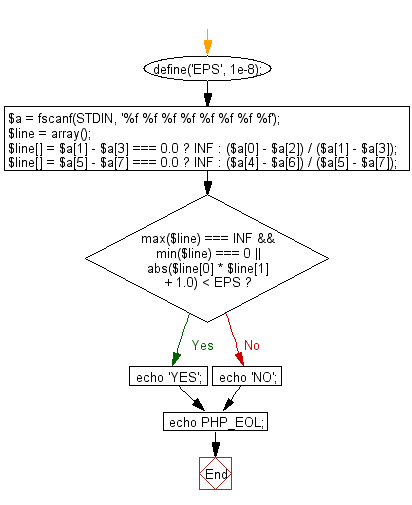﻿ PHP Exercise: Test whether AB and CD are orthogonal or not - w3resource

# PHP Exercises: Test whether AB and CD are orthogonal or not

## PHP: Exercise-68 with Solution

There are four different points on a plane, P(xp,yp), Q(xq, yq), R(xr, yr) and S(xs, ys). Write a PHP program to test whether AB and CD are orthogonal or not.
xp,yp, xq, yq, xr, yr, xs and ys are -100 to 100 respectively and each value can be up to 5 digits after the decimal point It is given as a real number including the number of.

Sample Solution: -

PHP Code:

``````<?php
define('EPS', 1e-8);
\$a = fscanf(STDIN, '%f %f %f %f %f %f %f %f');
\$line = array();
\$line[] = \$a - \$a === 0.0 ? INF : (\$a - \$a) / (\$a - \$a);
\$line[] = \$a - \$a === 0.0 ? INF : (\$a - \$a) / (\$a - \$a);
if (max(\$line) === INF && min(\$line) === 0 || abs(\$line * \$line + 1.0) < EPS) {
echo 'Orthogonal';
} else {
echo 'Not orthogonal';
}
echo PHP_EOL;
?>
```
```

Sample Input:
3.5 4.5 2.5 -1.5 3.5 1.0 0.0 4.5

Sample Output:

`Not orthogonal`

Flowchart:PHP Code Editor:

Have another way to solve this solution? Contribute your code (and comments) through Disqus.

What is the difficulty level of this exercise?

Test your Programming skills with w3resource's quiz.

﻿

## PHP: Tips of the Day

Returns all elements in an array except for the first one

Example:

```<?php
function tips_tail(\$items)
{
return count(\$items) > 1 ? array_slice(\$items, 1) : \$items;
}

print_r(tips_tail([1, 5, 7]));
?>
```

Output:

```Array
(
 => 5
 => 7
)
```